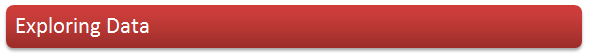Looking At Data
Stem-And-Leaf Diagrams
Types Of Data
Measures of Central Tendency
Frequency Distributions
Grouped Data
Linear Coding
Key Points of This Module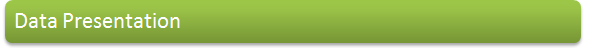Bar Charts and Vertical Line Charts
Pie Charts
Histograms
Meausres of Central Tendency and of Spread Using Quartiles
Cumulative Frequency Curves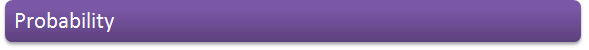Estimating Probability
Expectation
The Probability of Either One Event or Another
The Probability of Events From Two Trials
Conditional Probability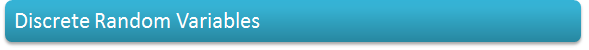Discrete Random Variables
Expectation and Variance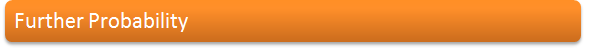Factorials
Permutations
Combinations
The Binomial Coefficients nCr
Calculating Probabilities In Less Simple Cases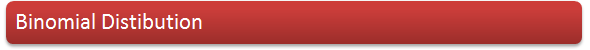The Binomial Distribution
The Expectation of B(n, p)
Using The Binomial Distribution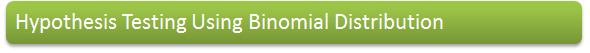Defining Terms
Hypothesis Testing Checklist
Choosing The Significance Level
Critical Values and Critical Regions
1-Tail and 2-Tail Tests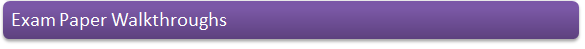January 2008